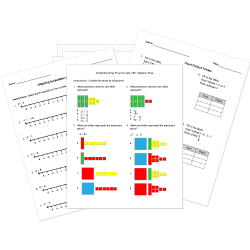Looking for Algebra worksheets?
Check out our pre-made Algebra worksheets!
 Tweet##### Browse Questions
• Arts (658)
• English Language Arts (3081)
• English as a Second Language ESL (1429)
• Health and Medicine (721)
• Life Skills (476)
• Math (2471)

• ### Systems of Equations

• #### Trigonometry

• Physical Education (420)
• Science (7122)
• Social Studies (2157)
• Study Skills and Strategies (13)
• Technology (485)
• Vocational Education (1000)

You can create printable tests and worksheets from these Grade 10 Nonlinear Equations and Functions questions! Select one or more questions using the checkboxes above each question. Then click the add selected questions to a test button before moving to another page.

Previous Next
Grade 10 Nonlinear Equations and Functions CCSS: HSA-REI.A.2
Solve for $y$.
$(20y-12)/(4y)=4$
1. $3$
2. $5$
3. $2$
4. $-1$
Grade 10 Nonlinear Equations and Functions CCSS: HSA-REI.A.2
Solve for $t$.
$sqrt(5t+11)=6$
1. $11$
2. $46/55$
3. $10$
4. $5$
Grade 10 Nonlinear Equations and Functions CCSS: HSA-REI.A.2
Grade 10 Nonlinear Equations and Functions
Grade 10 Nonlinear Equations and Functions CCSS: HSA-REI.A.2
Solve for $x$.
$(2x-3)/(x-5)=3$
1. $12$
2. $21/7$
3. $2$
4. $2/3$
Grade 10 Nonlinear Equations and Functions CCSS: HSA-REI.A.2
Solve for $x$.
$sqrt(7x)/x=x/7$
1. $1$
2. $1/7$
3. $7$
4. $-1$
Grade 10 Nonlinear Equations and Functions
If $4x^3 = - 1/54, x =$
1. $- 1/3$
2. $- 1/6$
3. $- 1/9$
4. $- 1/12$
Grade 10 Nonlinear Equations and Functions
A circle with equation $(x+4)^2 + (y-3)^2 = 16$ is shifted 5 units to the right. What is the equation of the new circle?
1. $(x-1)^2+ (y - 3)^2 = 16$
2. $(x+1)^2+ (y + 3)^2 = 16$
3. $(x-1)^2+ (y - 8)^2 = 16$
4. $(x-5)^2+ (y - 5)^2 = 16$
Grade 10 Nonlinear Equations and Functions
The equation $(x+1)^2-1=x(x+2)$ is an example of
2. an identity.
3. a conditional equation.
4. a linear equation.
Grade 10 Nonlinear Equations and Functions CCSS: HSA-REI.A.2
Grade 10 Nonlinear Equations and Functions
Find the real-number solutions of the equation:
$x^4-14x^2+45=0$
1. $+-3, +-sqrt(5)$
2. $+-3, +-5i$
3. $9, 5$
4. No solution
Grade 10 Nonlinear Equations and Functions
Find the real number solutions of the equation:
$x^3+2x^2-25x-50=0$
1. $-5, 5, 0$
2. $-2, 2, 25$
3. $-5, -2, 5$
4. No solution
Grade 10 Nonlinear Equations and Functions
A circle is tangent to the x-axis at (5,0) and tangent to the y-axis at (0,-5). Determine the equation of this circle.
1. $(x-5)^2+ (y + 5)^2 = 5$
2. $(x-5)^2+ (y - 5)^2 = 5$
3. $(x-5)^2+ (y - 5)^2 = 25$
4. $(x-5)^2+ (y + 5)^2 = 25$
Grade 10 Nonlinear Equations and Functions CCSS: HSA-REI.A.2
If $(x-1)/x = 4$, then $x =$
1. $3$
2. $-3$
3. $-1/6$
4. $-1/3$
Grade 10 Nonlinear Equations and Functions
Grade 10 Nonlinear Equations and Functions CCSS: HSA-REI.A.2
Find the value of $y$ in $1/(4y) - 1/(3y)=3/16$ .
1. $4/9$
2. $9/4$
3. $-9/4$
4. $-4/9$
Grade 10 Nonlinear Equations and Functions
Previous Next
You need to have at least 5 reputation to vote a question down. Learn How To Earn Badges.